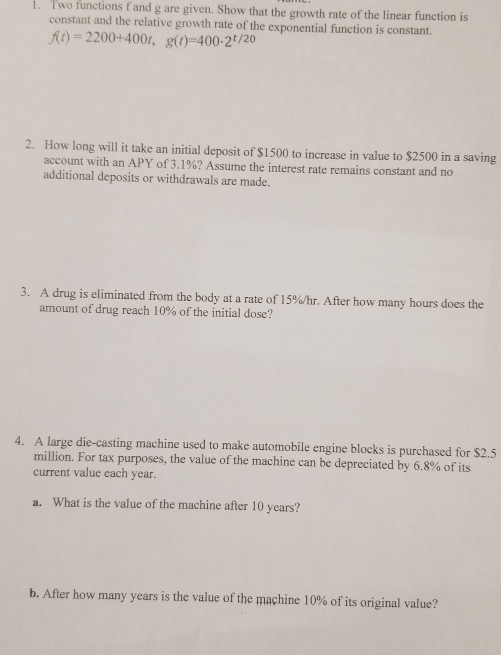Home / Answered Questions / Other / 1-two-functions-and-g-are-given-show-that-the-growth-rate-of-the-linear-function-is-constant-and-the-aw175

# (Solved): 1. Two Functions And G Are Given. Show That The Growth Rate Of The Linear Function Is Constant And T...1. Two functions and g are given. Show that the growth rate of the linear function is constant and the relative growth rate of the exponential function is constant. ) = 2200+4001, g(0)-400-20/20 2. How long will it take an initial deposit of \$1500 to increase in value to \$2500 in a saving account with an APY of 3.1%? Assume the interest rate remains constant and no additional deposits or withdrawals are made. 3. A drug is eliminated from the body at a rate of 15%/hr. After how many hours does the amount of drug reach 10% of the initial dose? 4. A large die-casting machine used to make automobile engine blocks is purchased for \$2.5 million. For tax purposes, the value of the machine can be depreciated by 6.8% of its current value each year. a. What is the value of the machine after 10 years? b. After how many years is the value of the machine 10% of its original value?

We have an Answer from Expert